Theory and Modern Applications

# Existence of solutions for discrete fractional boundary value problems with a p-Laplacian operator

## Abstract

This paper is concerned with the existence of solutions to a discrete fractional boundary value problem with a p-Laplacian operator. Under certain nonlinear growth conditions of the nonlinearity, the existence result is established by using Schaefer’s fixed point theorem. Additionally, a representative example is presented to illustrate the effectiveness of the main result.

MSC:26A33, 39A05, 39A12.

## 1 Introduction

For any number $a\in \mathbb{R}$ and each interval I of $\mathbb{R}$, we denote ${\mathrm{N}}_{a}=\left\{a,a+1,a+2,\dots \right\}$ and ${\mathrm{I}}_{{\mathrm{N}}_{a}}=\mathrm{I}\cap {\mathrm{N}}_{a}$ throughout this paper. It is also worth noting that, in what follows, we appeal to the convention that ${\sum }_{s=k}^{k-1}u\left(s\right)=0$ for any $k\in {\mathrm{N}}_{a}$, where u is a function defined on ${\mathrm{N}}_{a}$.

In this paper, we will consider the following discrete fractional boundary value problem with a p-Laplacian operator:

$\left\{\begin{array}{c}{\mathrm{\Delta }}_{C}^{\beta }\left[{\varphi }_{p}\left({\mathrm{\Delta }}_{C}^{\alpha }u\right)\right]\left(t\right)=f\left(t+\alpha +\beta -1,u\left(t+\alpha +\beta -1\right)\right),\phantom{\rule{1em}{0ex}}t\in {\left[0,b\right]}_{{\mathrm{N}}_{0}},\hfill \\ {\mathrm{\Delta }}_{C}^{\alpha }u\left(t\right){|}_{t=\beta -1}+{\mathrm{\Delta }}_{C}^{\alpha }u\left(t\right){|}_{t=\beta +b}=0,\hfill \\ u\left(\alpha +\beta -2\right)+u\left(\alpha +\beta +b\right)=0,\hfill \end{array}$
(1.1)

where $0<\alpha$, $\beta \le 1$, $1<\alpha +\beta \le 2$, $b\in {\mathrm{N}}_{1}$, ${\mathrm{\Delta }}_{C}^{\beta }$ and ${\mathrm{\Delta }}_{C}^{\alpha }$ denote the Caputo fractional differences of order α and β respectively, $f:{\left[\alpha +\beta -1,b+\alpha +\beta -1\right]}_{{\mathrm{N}}_{\alpha +\beta -1}}×\mathbb{R}\to \mathbb{R}$ is a continuous function and ${\varphi }_{p}$ is the p-Laplacian operator, that is, ${\varphi }_{p}\left(u\right)={|u|}^{p-2}u$, $p>1$. Obviously, ${\varphi }_{p}$ is invertible and its inverse operator is ${\varphi }_{q}$, where $q>1$ is a constant such that $1/p+1/q=1$.

The continuous fractional calculus has received increasing attention within the last ten years or so, and the theory of fractional differential equations has been a new important mathematical branch due to its extensive applications in various fields of science, such as physics, mechanics, chemistry, engineering, etc. For more details, see  and references therein. Although the discrete fractional calculus has seen slower progress, within the recent several years, a lot of papers have appeared; see . For example, a recent paper by Atıcı and Eloe  explores a discrete fractional conjugate boundary value problem with the Riemann-Liouville fractional difference. To the best of our knowledge, this is a pioneering work on discussing boundary value problems in discrete fractional calculus. After that, Goodrich studied discrete fractional boundary value problems involving the Riemann-Liouville fractional difference intensively and obtained a series of excellent results; see . Particularly note that Abdeljawad introduced the conception of Caputo fractional difference and presented some useful properties of it in .

p-Laplacian boundary value problems for ordinary differential equations, finite difference equations and dynamic equations have been studied extensively, but there are few papers dealing with the fractional p-Laplacian boundary value problems, besides , especially for discrete fractional p-Laplacian boundary value problems involving Caputo fractional differences.

Motivated by the aforementioned works, we will consider the existence of solutions to the discrete fractional boundary value problem (1.1) and establish the sufficient conditions for the existence of at least one solution to it by using Schaefer’s fixed point theorem.

The remainder of this paper is organized as follows. Section 2 preliminarily provides some necessary background material for the theory of discrete fractional calculus. In Section 3, the main existence result for problem (1.1) is established with the help of Schaefer’s fixed point theorem. Finally, in Section 4, a concrete example is provided to illustrate the possible application of the established analytical result.

## 2 Preliminaries

For convenience, we first present here some necessary basic definitions on discrete fractional calculus.

Definition 2.1 

For any t and ν, the falling factorial function is defined as

${t}^{\underline{\nu }}=\frac{\mathrm{\Gamma }\left(t+1\right)}{\mathrm{\Gamma }\left(t+1-\nu \right)}$

provided that the right-hand side is well defined. We appeal to the convention that if $t+1-\nu$ is a pole of the Gamma function and $t+1$ is not a pole, then ${t}^{\underline{\nu }}=0$.

Definition 2.2 

For any t and ν, the rising factorial function is defined as

${t}^{\overline{\nu }}=\frac{\mathrm{\Gamma }\left(t+\nu \right)}{\mathrm{\Gamma }\left(t\right)}$

provided that the right-hand side is well defined. We employ the convention that if t is a pole of the Gamma function and $t+\nu$ is not a pole, then ${t}^{\overline{\nu }}=0$.

Definition 2.3 

The ν-th fractional sum of a function $f:{\mathrm{N}}_{a}\to \mathbb{R}$, for $\nu >0$, is defined by

${\mathrm{\Delta }}^{-\nu }f\left(t\right)=\frac{1}{\mathrm{\Gamma }\left(\nu \right)}\sum _{s=a}^{t-\nu }{\left(t-s-1\right)}^{\underline{\nu -1}}f\left(s\right),\phantom{\rule{1em}{0ex}}\mathrm{\forall }t\in {\mathrm{N}}_{a+\nu }.$

Definition 2.4 

The ν-order Caputo fractional difference of a function $f:{\mathrm{N}}_{a}\to \mathbb{R}$, for $\nu >0$, $\nu \notin \mathrm{N}$, is defined by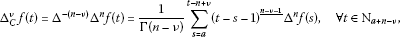where n is the smallest integer greater than or equal to ν and ${\mathrm{\Delta }}^{n}$ is the n-th order forward difference operator. If $\nu =n\in \mathrm{N}$, then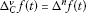.

Next, we present here several lemmas which will be important in the sequel.

Lemma 2.1 

Assume that $\nu >0$ and f is defined on ${\mathrm{N}}_{a}$. Then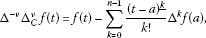where n is the smallest integer greater than or equal to ν.

Lemma 2.2 Let n be a positive integer. Then

${\left(t-a\right)}^{\underline{n}}=\sum _{k=0}^{n}\left(\begin{array}{c}n\\ k\end{array}\right){\left(-1\right)}^{k}{a}^{\overline{k}}{t}^{\underline{n-k}},\phantom{\rule{1em}{0ex}}t\in {\mathrm{N}}_{a}.$
(2.1)

Proof The proof is by induction. For $n=1$, (2.1) is the same as

${\left(t-a\right)}^{\underline{1}}=t-a.$

Assume that (2.1) is true for $n=m$, that is

${\left(t-a\right)}^{\underline{m}}=\sum _{k=0}^{m}\left(\begin{array}{c}m\\ k\end{array}\right){\left(-1\right)}^{k}{a}^{\overline{k}}{t}^{\underline{m-k}},\phantom{\rule{1em}{0ex}}t\in {\mathrm{N}}_{a}$

holds. So, for $n=m+1$,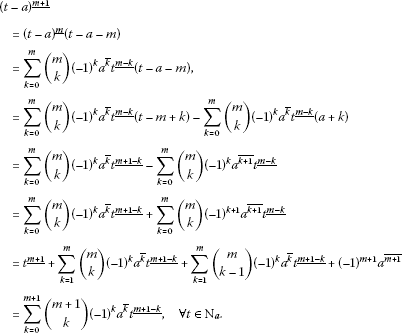Therefore, (2.1) also holds for $n=m+1$, which implies that (2.1) holds for any positive integer. □

In view of Lemma 2.1 and Lemma 2.2, the following fact is obvious.

Lemma 2.3 Assume that $\nu >0$ and f is defined on ${\mathrm{N}}_{a}$. Then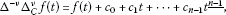where ${c}_{i}\in \mathbb{R}$, $i=1,2,\dots ,n-1$, and n is the smallest integer greater than or equal to ν.

Finally, we need the following additional lemma that will be used in Section 3 of this paper.

Lemma 2.4 

Let $\nu \in \mathbb{R}$ and $t,s\in \mathbb{R}$ such that ${\left(t-s\right)}^{\underline{\nu }}$ is well defined. Then

${\mathrm{\Delta }}_{s}{\left(t-s\right)}^{\underline{\nu }}=-\nu {\left(t-s-1\right)}^{\underline{\nu -1}}.$

## 3 Existence result

In this section, we will establish the existence of at least one solution for problem (1.1). To accomplish this, we first state and prove the following result which is of particular importance in what follows.

Lemma 3.1 Let $h:{\left[\alpha +\beta -1,\alpha +\beta -1+b\right]}_{{\mathrm{N}}_{\alpha +\beta -1}}\to \mathbb{R}$ be given. The unique solution of

$\left\{\begin{array}{c}{\mathrm{\Delta }}_{C}^{\beta }\left[{\varphi }_{p}\left({\mathrm{\Delta }}_{C}^{\alpha }u\right)\right]\left(t\right)=h\left(t+\alpha +\beta -1\right),\phantom{\rule{1em}{0ex}}t\in {\left[0,b\right]}_{{\mathrm{N}}_{0}},\hfill \\ {\mathrm{\Delta }}_{C}^{\alpha }u\left(t\right){|}_{t=\beta -1}+{\mathrm{\Delta }}_{C}^{\alpha }u\left(t\right){|}_{t=\beta +b}=0,\hfill \\ u\left(\alpha +\beta -2\right)+u\left(\alpha +\beta +b\right)=0,\hfill \end{array}$
(3.1)

is

$\begin{array}{rcl}u\left(t\right)& =& \frac{1}{\mathrm{\Gamma }\left(\alpha \right)}\sum _{s=\beta -1}^{t-\alpha }{\left(t-s-1\right)}^{\underline{\alpha -1}}{\varphi }_{q}\left(\frac{1}{\mathrm{\Gamma }\left(\beta \right)}\sum _{\tau =0}^{s-\beta }{\left(s-\tau -1\right)}^{\underline{\beta -1}}h\left(\tau +\alpha +\beta -1\right)\\ -\frac{1}{2\mathrm{\Gamma }\left(\beta \right)}\sum _{\tau =0}^{b}{\left(\beta +b-\tau -1\right)}^{\underline{\beta -1}}h\left(\tau +\alpha +\beta -1\right)\right)\\ -\frac{1}{2\mathrm{\Gamma }\left(\alpha \right)}\sum _{s=\beta -1}^{\beta +b}{\left(\alpha +\beta +b-s-1\right)}^{\underline{\alpha -1}}{\varphi }_{q}\left(\frac{1}{\mathrm{\Gamma }\left(\beta \right)}\sum _{\tau =0}^{s-\beta }{\left(s-\tau -1\right)}^{\underline{\beta -1}}h\left(\tau +\alpha +\beta -1\right)\\ -\frac{1}{2\mathrm{\Gamma }\left(\beta \right)}\sum _{\tau =0}^{b}{\left(\beta +b-\tau -1\right)}^{\underline{\beta -1}}h\left(\tau +\alpha +\beta -1\right)\right),\\ t\in {\left[\alpha +\beta -2,\alpha +\beta +b\right]}_{{\mathrm{N}}_{\alpha +\beta -2}}.\end{array}$

Proof Suppose that $u\left(t\right)$ satisfies the equation of problem (3.1), then Lemma 2.3 implies that

${\varphi }_{p}\left({\mathrm{\Delta }}_{C}^{\alpha }u\left(t\right)\right)=\frac{1}{\mathrm{\Gamma }\left(\beta \right)}\sum _{s=0}^{t-\beta }{\left(t-s-1\right)}^{\underline{\beta -1}}h\left(s+\alpha +\beta -1\right)+{c}_{0},$

for some ${c}_{0}\in \mathbb{R}$, $t\in {\left[\beta -1,\beta +b\right]}_{{\mathrm{N}}_{\beta -1}}$.

From the boundary condition ${\mathrm{\Delta }}_{C}^{\alpha }u\left(t\right){|}_{t=\beta -1}+{\mathrm{\Delta }}_{C}^{\alpha }u\left(t\right){|}_{t=\beta +b}=0$, one has

${c}_{0}=-\frac{1}{2\mathrm{\Gamma }\left(\beta \right)}\sum _{s=0}^{b}{\left(\beta +b-s-1\right)}^{\underline{\beta -1}}h\left(s+\alpha +\beta -1\right).$

Therefore, we have

$\begin{array}{rcl}u\left(t\right)& =& \frac{1}{\mathrm{\Gamma }\left(\alpha \right)}\sum _{s=\beta -1}^{t-\alpha }{\left(t-s-1\right)}^{\underline{\alpha -1}}{\varphi }_{q}\left(\frac{1}{\mathrm{\Gamma }\left(\beta \right)}\sum _{\tau =0}^{s-\beta }{\left(s-\tau -1\right)}^{\underline{\beta -1}}h\left(\tau +\alpha +\beta -1\right)\\ -\frac{1}{2\mathrm{\Gamma }\left(\beta \right)}\sum _{\tau =0}^{b}{\left(\beta +b-\tau -1\right)}^{\underline{\beta -1}}h\left(\tau +\alpha +\beta -1\right)\right)+{c}_{1},\end{array}$
(3.2)

where ${c}_{1}\in \mathbb{R}$, $t\in {\left[\alpha +\beta -2,\alpha +\beta +b\right]}_{{\mathrm{N}}_{\alpha +\beta -2}}$.

On the other hand, by condition $u\left(\alpha +\beta -2\right)+u\left(\alpha +\beta +b\right)=0$, we get

$\begin{array}{rcl}{c}_{1}& =& -\frac{1}{2\mathrm{\Gamma }\left(\alpha \right)}\sum _{s=\beta -1}^{\beta +b}{\left(\alpha +\beta +b-s-1\right)}^{\underline{\alpha -1}}{\varphi }_{q}\left(\frac{1}{\mathrm{\Gamma }\left(\beta \right)}\sum _{\tau =0}^{s-\beta }{\left(s-\tau -1\right)}^{\underline{\beta -1}}h\left(\tau +\alpha +\beta -1\right)\\ -\frac{1}{2\mathrm{\Gamma }\left(\beta \right)}\sum _{\tau =0}^{b}{\left(\beta +b-\tau -1\right)}^{\underline{\beta -1}}h\left(\tau +\alpha +\beta -1\right)\right).\end{array}$

Now, substitution of ${c}_{1}$ into (3.2) gives

$\begin{array}{rcl}u\left(t\right)& =& \frac{1}{\mathrm{\Gamma }\left(\alpha \right)}\sum _{s=\beta -1}^{t-\alpha }{\left(t-s-1\right)}^{\underline{\alpha -1}}{\varphi }_{q}\left(\frac{1}{\mathrm{\Gamma }\left(\beta \right)}\sum _{\tau =0}^{s-\beta }{\left(s-\tau -1\right)}^{\underline{\beta -1}}h\left(\tau +\alpha +\beta -1\right)\\ -\frac{1}{2\mathrm{\Gamma }\left(\beta \right)}\sum _{\tau =0}^{b}{\left(\beta +b-\tau -1\right)}^{\underline{\beta -1}}h\left(\tau +\alpha +\beta -1\right)\right)\\ -\frac{1}{2\mathrm{\Gamma }\left(\alpha \right)}\sum _{s=\beta -1}^{\beta +b}{\left(\alpha +\beta +b-s-1\right)}^{\underline{\alpha -1}}{\varphi }_{q}\left(\frac{1}{\mathrm{\Gamma }\left(\beta \right)}\sum _{\tau =0}^{s-\beta }{\left(s-\tau -1\right)}^{\underline{\beta -1}}h\left(\tau +\alpha +\beta -1\right)\\ -\frac{1}{2\mathrm{\Gamma }\left(\beta \right)}\sum _{\tau =0}^{b}{\left(\beta +b-\tau -1\right)}^{\underline{\beta -1}}h\left(\tau +\alpha +\beta -1\right)\right),\\ t\in {\left[\alpha +\beta -2,\alpha +\beta +b\right]}_{{\mathrm{N}}_{\alpha +\beta -2}}.\end{array}$

The proof is complete. □

To prove the main result, we need Schaefer’s fixed point theorem.

Lemma 3.2 

Let E be a normed linear space (possibly incomplete) and $\mathrm{\Phi }:E\to E$ be a compact operator. Suppose that the set

$S=\left\{x\in E|x=\lambda \mathrm{\Phi }x,\phantom{\rule{0.5em}{0ex}}\mathit{\text{for some}}\phantom{\rule{0.5em}{0ex}}\lambda \in \left(0,1\right)\right\}$

is bounded. Then Φ has a fixed point in E.

In order to use Schaefer’s fixed point theorem to solve the problem (1.1), we first define the following operator:

$\mathcal{F}:C{\left[\alpha +\beta -2,\alpha +\beta +b\right]}_{{\mathrm{N}}_{\alpha +\beta -2}}\to C{\left[\alpha +\beta -2,\alpha +\beta +b\right]}_{{\mathrm{N}}_{\alpha +\beta -2}}$

by

$\begin{array}{rcl}\mathcal{F}u\left(t\right)& =& \frac{1}{\mathrm{\Gamma }\left(\alpha \right)}\sum _{s=\beta -1}^{t-\alpha }{\left(t-s-1\right)}^{\underline{\alpha -1}}{\varphi }_{q}\left(\frac{1}{\mathrm{\Gamma }\left(\beta \right)}\sum _{\tau =0}^{s-\beta }{\left(s-\tau -1\right)}^{\underline{\beta -1}}\mathcal{N}u\left(\tau +\alpha +\beta -1\right)\\ -\frac{1}{2\mathrm{\Gamma }\left(\beta \right)}\sum _{\tau =0}^{b}{\left(\beta +b-\tau -1\right)}^{\underline{\beta -1}}\mathcal{N}u\left(\tau +\alpha +\beta -1\right)\right)\\ -\frac{1}{2\mathrm{\Gamma }\left(\alpha \right)}\sum _{s=\beta -1}^{\beta +b}{\left(\alpha +\beta +b-s-1\right)}^{\underline{\alpha -1}}\\ ×{\varphi }_{q}\left(\frac{1}{\mathrm{\Gamma }\left(\beta \right)}\sum _{\tau =0}^{s-\beta }{\left(s-\tau -1\right)}^{\underline{\beta -1}}\mathcal{N}u\left(\tau +\alpha +\beta -1\right)\\ -\frac{1}{2\mathrm{\Gamma }\left(\beta \right)}\sum _{\tau =0}^{b}{\left(\beta +b-\tau -1\right)}^{\underline{\beta -1}}\mathcal{N}u\left(\tau +\alpha +\beta -1\right)\right),\end{array}$

where $C{\left[\alpha +\beta -2,\alpha +\beta +b\right]}_{{\mathrm{N}}_{\alpha +\beta -2}}$ denotes the Banach space of all functions $u:{\left[\alpha +\beta -2,\alpha +\beta +b\right]}_{{\mathrm{N}}_{\alpha +\beta -2}}\to \mathbb{R}$ with the norm $\parallel u\parallel =max\left\{|u\left(t\right)|:t\in {\left[\alpha +\beta -2,\alpha +\beta +b\right]}_{{\mathrm{N}}_{\alpha +\beta -2}}\right\}$ and the operator

$\mathcal{N}:C{\left[\alpha +\beta -2,\alpha +\beta +b\right]}_{{\mathrm{N}}_{\alpha +\beta -2}}\to C{\left[\alpha +\beta -2,\alpha +\beta +b\right]}_{{\mathrm{N}}_{\alpha +\beta -2}}$

is defined by

$\mathcal{N}u\left(t\right)=f\left(t,u\left(t\right)\right),\phantom{\rule{1em}{0ex}}\mathrm{\forall }t\in {\left[\alpha +\beta -2,\alpha +\beta +b\right]}_{{\mathrm{N}}_{\alpha +\beta -2}}.$

It is easy to verify that the operator $\mathcal{F}$ is well defined, and the fixed points of the operator $\mathcal{F}$ are solutions of problem (1.1).

Now, the main result is stated as follows.

Theorem 3.1 Assume that there exist nonnegative functions $a,g\in C{\left[\alpha +\beta -2,\alpha +\beta +b\right]}_{{\mathrm{N}}_{\alpha +\beta -2}}$ such that

$|f\left(t,u\right)|\le a\left(t\right)+g\left(t\right){|u|}^{p-1},\phantom{\rule{1em}{0ex}}\mathrm{\forall }t\in {\left[\alpha +\beta -2,\alpha +\beta +b\right]}_{{\mathrm{N}}_{\alpha +\beta -2}},u\in \mathbb{R}.$
(3.3)

Then the problem (1.1) has at least one solution, provided that

${\left(3/2\right)}^{q}{\parallel g\parallel }^{q-1}\left(\prod _{i=1}^{b}{\left(1+\frac{\beta }{i}\right)}^{q-1}\right)\left(\prod _{i=1}^{b+1}\left(1+\frac{\alpha }{i}\right)\right)<1.$
(3.4)

Proof The proof will be divided into the following two steps.

Step 1: $\mathcal{F}:C{\left[\alpha +\beta -2,\alpha +\beta +b\right]}_{{\mathrm{N}}_{\alpha +\beta -2}}\to C{\left[\alpha +\beta -2,\alpha +\beta +b\right]}_{{\mathrm{N}}_{\alpha +\beta -2}}$ is completely continuous.

At first, in view of the continuity of f, it is easy to verify that $\mathcal{F}$ is continuous. Furthermore, it is not difficult to verify that $\mathcal{F}$ maps bounded sets into bounded sets and equi-continuous sets. Therefore, in the light of the well-known Arzelá-Ascoli theorem, we know that $\mathcal{F}$ is a compact operator.

Step 2: $\mathcal{F}$ a priori bounds.

Set

$S=\left\{u\in C{\left[\alpha +\beta -2,\alpha +\beta +b\right]}_{{\mathrm{N}}_{\alpha +\beta -2}}|u=\lambda \mathcal{F}u,\lambda \in \left(0,1\right)\right\}.$

Now, it remains to show that the set S is bounded.

For any $u\in S$, there exists a $\lambda \in \left(0,1\right)$ such that $u\left(t\right)=\lambda \mathcal{F}u\left(t\right)$. So, by (3.3), Lemma 2.4 and the monotonicity of ${s}^{\underline{\iota }}$, $\iota \in \left(0,1\right]$, we can obtain that

$\begin{array}{rcl}|u\left(t\right)|& =& \lambda |\mathcal{F}u\left(t\right)|\\ \le & \frac{1}{\mathrm{\Gamma }\left(\alpha \right)}\sum _{s=\beta -1}^{t-\alpha }{\left(t-s-1\right)}^{\underline{\alpha -1}}{\varphi }_{q}\left(\frac{1}{\mathrm{\Gamma }\left(\beta \right)}\sum _{\tau =0}^{s-\beta }{\left(s-\tau -1\right)}^{\underline{\beta -1}}|\mathcal{N}u\left(\tau +\alpha +\beta -1\right)|\\ +\frac{1}{2\mathrm{\Gamma }\left(\beta \right)}\sum _{\tau =0}^{b}{\left(\beta +b-\tau -1\right)}^{\underline{\beta -1}}|\mathcal{N}u\left(\tau +\alpha +\beta -1\right)|\right)\\ +\frac{1}{2\mathrm{\Gamma }\left(\alpha \right)}\sum _{s=\beta -1}^{\beta +b}{\left(\alpha +\beta +b-s-1\right)}^{\underline{\alpha -1}}\\ ×{\varphi }_{q}\left(\frac{1}{\mathrm{\Gamma }\left(\beta \right)}\sum _{\tau =0}^{s-\beta }{\left(s-\tau -1\right)}^{\underline{\beta -1}}|\mathcal{N}u\left(\tau +\alpha +\beta -1\right)|\\ +\frac{1}{2\mathrm{\Gamma }\left(\beta \right)}\sum _{\tau =0}^{b}{\left(\beta +b-\tau -1\right)}^{\underline{\beta -1}}|\mathcal{N}u\left(\tau +\alpha +\beta -1\right)|\right),\\ \le & \frac{1}{\mathrm{\Gamma }\left(\alpha \right)}\sum _{s=\beta -1}^{t-\alpha }{\left(t-s-1\right)}^{\underline{\alpha -1}}{\varphi }_{q}\left(\frac{\parallel a\parallel +\parallel g\parallel {\parallel u\parallel }^{p-1}}{\mathrm{\Gamma }\left(\beta \right)}\sum _{\tau =0}^{s-\beta }{\left(s-\tau -1\right)}^{\underline{\beta -1}}\\ +\frac{\parallel a\parallel +\parallel g\parallel {\parallel u\parallel }^{p-1}}{2\mathrm{\Gamma }\left(\beta \right)}\sum _{\tau =0}^{b}{\left(\beta +b-\tau -1\right)}^{\underline{\beta -1}}\right)\\ +\frac{1}{2\mathrm{\Gamma }\left(\alpha \right)}\sum _{s=\beta -1}^{\beta +b}{\left(\alpha +\beta +b-s-1\right)}^{\underline{\alpha -1}}{\varphi }_{q}\left(\frac{\parallel a\parallel +\parallel g\parallel {\parallel u\parallel }^{p-1}}{\mathrm{\Gamma }\left(\beta \right)}\sum _{\tau =0}^{s-\beta }{\left(s-\tau -1\right)}^{\underline{\beta -1}}\\ +\frac{\parallel a\parallel +\parallel g\parallel {\parallel u\parallel }^{p-1}}{2\mathrm{\Gamma }\left(\beta \right)}\sum _{\tau =0}^{b}{\left(\beta +b-\tau -1\right)}^{\underline{\beta -1}}\right)\\ =& \frac{1}{\mathrm{\Gamma }\left(\alpha \right)}\sum _{s=\beta -1}^{t-\alpha }{\left(t-s-1\right)}^{\underline{\alpha -1}}\\ ×{\varphi }_{q}\left(\frac{\left(\parallel a\parallel +\parallel g\parallel {\parallel u\parallel }^{p-1}\right){s}^{\underline{\beta }}}{\mathrm{\Gamma }\left(\beta +1\right)}+\frac{\left(\parallel a\parallel +\parallel g\parallel {\parallel u\parallel }^{p-1}\right){\left(\beta +b\right)}^{\underline{\beta }}}{2\mathrm{\Gamma }\left(\beta +1\right)}\right)\\ +\frac{1}{2\mathrm{\Gamma }\left(\alpha \right)}\sum _{s=\beta -1}^{\beta +b}{\left(\alpha +\beta +b-s-1\right)}^{\underline{\alpha -1}}{\varphi }_{q}\left(\frac{\left(\parallel a\parallel +\parallel g\parallel {\parallel u\parallel }^{p-1}\right){s}^{\underline{\beta }}}{\mathrm{\Gamma }\left(\beta +1\right)}\\ +\frac{\left(\parallel a\parallel +\parallel g\parallel {\parallel u\parallel }^{p-1}\right){\left(\beta +b\right)}^{\underline{\beta }}}{2\mathrm{\Gamma }\left(\beta +1\right)}\right)\\ \le & {\varphi }_{q}\left(\frac{3{\left(\beta +b\right)}^{\underline{\beta }}\left(\parallel a\parallel +\parallel g\parallel {\parallel u\parallel }^{p-1}\right)}{2\mathrm{\Gamma }\left(\beta +1\right)}\right)\left\{\frac{1}{\mathrm{\Gamma }\left(\alpha \right)}\sum _{s=\beta -1}^{t-\alpha }{\left(t-s-1\right)}^{\underline{\alpha -1}}\\ +\frac{1}{2\mathrm{\Gamma }\left(\alpha \right)}\sum _{s=\beta -1}^{\beta +b}{\left(\alpha +\beta +b-s-1\right)}^{\underline{\alpha -1}}\right\}\\ \le & \frac{{3}^{q-1}{\left({\left(\beta +b\right)}^{\underline{\beta }}\right)}^{q-1}{\left(\parallel a\parallel +\parallel g\parallel {\parallel u\parallel }^{p-1}\right)}^{q-1}}{{2}^{q-1}{\left(\mathrm{\Gamma }\left(\beta +1\right)\right)}^{q-1}}\frac{3{\left(\alpha +b+1\right)}^{\underline{\alpha }}}{2\mathrm{\Gamma }\left(\alpha +1\right)}\\ \le & {\left(\frac{3}{2}\right)}^{q}{\left(\frac{{\left(\beta +b\right)}^{\underline{\beta }}}{\mathrm{\Gamma }\left(\beta +1\right)}\right)}^{q-1}\frac{{\left(\alpha +\beta +1\right)}^{\underline{\alpha }}}{\mathrm{\Gamma }\left(\alpha +1\right)}{\left(\parallel a\parallel +\parallel g\parallel {\parallel u\parallel }^{p-1}\right)}^{q-1}\\ =& {\left(\frac{3}{2}\right)}^{q}{\left(\frac{\mathrm{\Gamma }\left(\beta +b+1\right)}{\mathrm{\Gamma }\left(\beta +1\right)\mathrm{\Gamma }\left(b+1\right)}\right)}^{q-1}\frac{\mathrm{\Gamma }\left(\alpha +b+2\right)}{\mathrm{\Gamma }\left(\alpha +1\right)\mathrm{\Gamma }\left(b+2\right)}{\left(\parallel a\parallel +\parallel g\parallel {\parallel u\parallel }^{p-1}\right)}^{q-1}\\ =& {\left(\frac{3}{2}\right)}^{q}\left(\prod _{i=1}^{b}{\left(1+\frac{\beta }{i}\right)}^{q-1}\right)\left(\prod _{i=1}^{b+1}\left(1+\frac{\alpha }{i}\right)\right){\left(\parallel a\parallel +\parallel g\parallel {\parallel u\parallel }^{p-1}\right)}^{q-1}.\end{array}$
(3.5)

Here we will consider the following two cases: (1) $\parallel g\parallel =0$ or (2) $\parallel g\parallel \ne 0$.

Case 1: Suppose that $\parallel g\parallel =0$. Then it is evident that the set S is bounded from (3.5).

Case 2: Suppose that $\parallel g\parallel \ne 0$. It also follows from (3.5) that

${\parallel u\parallel }^{p-1}\le {\left\{{\left(\frac{3}{2}\right)}^{q}{\parallel g\parallel }^{q-1}\left(\prod _{i=1}^{b}{\left(1+\frac{\beta }{i}\right)}^{q-1}\right)\left(\prod _{i=1}^{b+1}\left(1+\frac{\alpha }{i}\right)\right)\right\}}^{p-1}\left(\frac{\parallel a\parallel }{\parallel g\parallel }+{\parallel u\parallel }^{p-1}\right).$
(3.6)

By virtue of (3.4) and (3.6), it is obvious that there exists a constant $M>0$ such that

$\parallel u\parallel \le M.$

Consequently, in both Case 1 and Case 2, we have proved that the set S is bounded.

Then, we can see that $\mathcal{F}$ satisfies all conditions of Schaefer’s fixed point theorem. Thus, we approach a conclusion that $\mathcal{F}$ has at least one fixed point which is the solution of problem (1.1). The proof is complete. □

## 4 An illustrative example

In this section, we will illustrate the possible application of the above established analytical result with a concrete example.

Example 4.1 Consider the following discrete fractional boundary value problem:

$\left\{\begin{array}{c}{\mathrm{\Delta }}_{C}^{1/2}\left[{\varphi }_{3}\left({\mathrm{\Delta }}_{C}^{2/3}u\right)\right]\left(t\right)=\frac{1}{100}{u}^{2}\left(t+1/6\right)+sin\left(t+1/6\right),\phantom{\rule{1em}{0ex}}t\in {\left[0,2\right]}_{{\mathrm{N}}_{0}},\hfill \\ {\mathrm{\Delta }}_{C}^{2/3}u\left(t\right){|}_{t=-1/2}+{\mathrm{\Delta }}_{C}^{2/3}u\left(t\right){|}_{t=5/2}=0,\hfill \\ u\left(-5/6\right)+u\left(19/6\right)=0.\hfill \end{array}$
(4.1)

Corresponding to problem (1.1), we have $p=3$, $q=\frac{3}{2}$, $\alpha =\frac{2}{3}$, $\beta =\frac{1}{2}$ and

$f\left(t,u\right)=\frac{1}{100}{u}^{2}+sint,\phantom{\rule{1em}{0ex}}t\in {\left[1/6,13/6\right]}_{{\mathrm{N}}_{1/6}},u\in \mathbb{R}.$

Choose $a\left(t\right)=1$, $g\left(t\right)=\frac{1}{100}$. By a simple calculation, we can obtain

${\left(\frac{3}{2}\right)}^{\frac{3}{2}}{\left(\frac{1}{100}\right)}^{\frac{1}{2}}\left(\prod _{i=1}^{2}{\left(1+\frac{1}{2i}\right)}^{\frac{1}{2}}\right)\left(\prod _{i=1}^{3}\left(1+\frac{2}{3i}\right)\right)\approx 0.6832<1.$

Obviously, problem (4.1) satisfies all assumptions of Theorem 3.1. Hence, we can conclude that problem (4.1) has at least one solution.

## References

1. Samko S, Kilbas A, Marichev O: Fractional Integrals and Derivatives: Theory and Applications. Gordon & Breach, Yverdon; 1993.

2. Glöckle W, Nonnenmacher T: A fractional calculus approach to self-similar protein dynamics. Biophys. J. 1995, 68(1):46–53. 10.1016/S0006-3495(95)80157-8

3. Metzler R, Schick W, Kilian H, Nonnenmacher T: Relaxation in filled polymers: a fractional calculus approach. J. Chem. Phys. 1995, 103(16):7180–7186. 10.1063/1.470346

4. Podlubny I Mathematics in Science and Engineering 198. In Fractional Differential Equations. Academic Press, San Diego; 1999.

5. Hilfer R: Applications of Fractional Calculus in Physics. World Scientific, Singapore; 2000.

6. Kilbas A, Trujillo J: Differential equations of fractional order: methods, results and problems-I. Appl. Anal. 2001, 78(1–2):153–192. 10.1080/00036810108840931

7. Kilbas A, Trujillo J: Differential equations of fractional order: methods, results and problems-II. Appl. Anal. 2002, 81(2):435–493. 10.1080/0003681021000022032

8. Sabatier J, Agrawal O, Machado Tenreiro J: Advances in Fractional Calculus: Theoretical Developments and Applications in Physics and Engineering. Springer, Dordrecht; 2007.

9. Frederico G, Torres D: Fractional conservation laws in optimal control theory. Nonlinear Dyn. 2008, 53(3):215–222. 10.1007/s11071-007-9309-z

10. Zhang S: The existence of a positive solution for a nonlinear fractional differential equation. J. Math. Anal. Appl. 2000, 252(2):804–812. 10.1006/jmaa.2000.7123

11. Zhang S: Existence of positive solution for some class of nonlinear fractional differential equations. J. Math. Anal. Appl. 2003, 278(1):136–148. 10.1016/S0022-247X(02)00583-8

12. Bai Z, Lü H: Positive solutions for boundary value problem of nonlinear fractional differential equation. J. Math. Anal. Appl. 2005, 311(2):495–505. 10.1016/j.jmaa.2005.02.052

13. Bai C: Triple positive solutions for a boundary value problem of nonlinear fractional differential equation. Electron. J. Qual. Theory Differ. Equ. 2008, 2008(24):1–10.

14. Xu X, Jiang D, Yuan C: Multiple positive solutions for the boundary value problem of a nonlinear fractional differential equation. Nonlinear Anal. TMA 2009, 71(10):4678–4688.

15. Atıcı F, Eloe P: A transform method in discrete fractional calculus. Int. J. Differ. Equ. 2007, 2(2):165–176.

16. Atıcı F, Eloe P: Initial value problems in discrete fractional calculus. Proc. Am. Math. Soc. 2009, 137(3):981–989.

17. Atıcı F, Eloe P: Discrete fractional calculus with the nabla operator. Electron. J. Qual. Theory Differ. Equ. 2009, 2009(3):1–12.

18. Atıcı F, Şengül S: Modeling with fractional difference equations. J. Math. Anal. Appl. 2010, 369(1):1–9. 10.1016/j.jmaa.2010.02.009

19. Atıcı F, Eloe P: Two-point boundary value problems for finite fractional difference equations. J. Differ. Equ. Appl. 2011, 17(4):445–456. 10.1080/10236190903029241

20. Goodrich C: Solutions to a discrete right-focal fractional boundary value problem. Int. J. Differ. Equ. 2010, 5(2):195–216.

21. Goodrich C: Continuity of solutions to discrete fractional initial value problems. Comput. Math. Appl. 2010, 59(11):3489–3499. 10.1016/j.camwa.2010.03.040

22. Goodrich C: Existence and uniqueness of solutions to a fractional difference equation with nonlocal conditions. Comput. Math. Appl. 2011, 61(2):191–202. 10.1016/j.camwa.2010.10.041

23. Goodrich C: Existence of a positive solution to a system of discrete fractional boundary value problems. Appl. Math. Comput. 2011, 217(9):4740–4753. 10.1016/j.amc.2010.11.029

24. Goodrich C: On a discrete fractional three-point boundary value problem. J. Differ. Equ. Appl. 2012, 18(3):397–415. 10.1080/10236198.2010.503240

25. Goodrich C: On discrete sequential fractional boundary value problems. J. Math. Anal. Appl. 2012, 385(1):111–124. 10.1016/j.jmaa.2011.06.022

26. Chen F, Luo X, Zhou Y: Existence results for nonlinear fractional difference equation. Adv. Differ. Equ. 2011., 2011: Article ID 713201

27. Bastos N, Ferreira R, Torres D: Discrete-time fractional variational problems. Signal Process. 2011, 91(3):513–524. 10.1016/j.sigpro.2010.05.001

28. Abdeljawad T: On Riemann and Caputo fractional differences. Comput. Math. Appl. 2011, 62(3):1602–1611. 10.1016/j.camwa.2011.03.036

29. Chen F: Fixed points and asymptotic stability of nonlinear fractional difference equations. Electron. J. Qual. Theory Differ. Equ. 2011, 2011(39):1–18.

30. Ferreira R: A discrete fractional Gronwall inequality. Proc. Am. Math. Soc. 2012, 140(5):1605–1612. 10.1090/S0002-9939-2012-11533-3

31. Ferreira R, Torres D: Fractional h -difference equations arising from the calculus of variations. Appl. Anal. Discrete Math. 2011, 5(1):110–121. 10.2298/AADM110131002F

32. Holm M: Sum and difference compositions in discrete fractional calculus. CUBO 2011, 13(3):153–184. 10.4067/S0719-06462011000300009

33. Abdeljawad T, Jarad F, Baleanu D: A semigroup-like property for discrete Mittag-Leffler functions. Adv. Differ. Equ. 2012., 2012: Article ID 72

34. Jarad F, Abdeljawad T, Baleanu D, Bicen K: On the stability of some discrete fractional non-autonomous systems. Abstr. Appl. Anal. 2012., 2012: Article ID 476581

35. Abdeljawad T, Baleanu D: Fractional differences and integration by parts. J. Comput. Anal. Appl. 2011, 13(3):574–582.

36. Wang J, Xiang H, Liu Z: Positive solutions for three-point boundary value problems of nonlinear fractional differential equations with p -Laplacian. Far East J. Appl. Math. 2009, 37(1):33–47.

37. Wang J, Xiang H: Upper and lower solutions method for a class of singular fractional boundary value problems with p -Laplacian operator. Abstr. Appl. Anal. 2010., 2010: Article ID 971824

38. Cai G: Positive solutions for boundary value problem for fractional differential equation with p -Laplacian operator. Bound. Value Probl. 2012., 2012: Article ID 18

39. Miller KS, Ross B: Fractional difference calculus. Proceedings of the International Symposium on Univalent Functions, Fractional Calculus and Their Applications 1989, 139–152.

40. Lloyd N: Degree Theory. Cambridge University Press, Cambridge; 1978.

## Acknowledgements

The author would like to express his thanks to the referees for their helpful comments and suggestions. This work was supported by the Longdong University Grant XYZK-1010 and XYZK-1007.

## Author information

Authors

### Corresponding author

Correspondence to Weidong Lv.

### Competing interests

The author declares that he has no competing interests.

## Rights and permissions

Reprints and Permissions

Lv, W. Existence of solutions for discrete fractional boundary value problems with a p-Laplacian operator. Adv Differ Equ 2012, 163 (2012). https://doi.org/10.1186/1687-1847-2012-163

• Accepted:

• Published:

• DOI: https://doi.org/10.1186/1687-1847-2012-163

### Keywords

• discrete fractional boundary value problem
• p-Laplacian operator
• existence
• Schaefer’s fixed point theorem